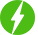# Implementation of various modulation techniques using MATLAB

1

Implementation of various modulation techniques using MATLAB, Learn analog and digital modulation techniques implementation using MATLAB.

## Course Description

This course is basically designed to demonstrate students how to use MATLAB in implementing different analog and digital modulation techniques used in communication. The course begin with introduction to electronics communication different concepts related to communication is explained along with the block diagram of analog and digital communication .There is discussion on types of communications systems – Analog communication and Digital communication. Different types of analog modulation-Amplitude modulation and frequency modulation and digital modulation techniques-amplitude shift keying, frequency shift keying and binary phase shift keying is explained in detailed .There is short introduction of MATLAB discussing different windows present in MATLAB, basic function used in MATLAB and how to write code in MATLAB. The MATLAB code for amplitude modulation and frequency modulation from Analog modulation and frequency shift keying and binary phase shift keying from digital communication is explained. Finally there is implementation of all this modulation techniques on MATLAB and verification of output waveforms for the same. For Amplitude modulation we are generating waveforms for modulation index greater than one and less than one condition. After completion of this course students get knowledge of different modulation techniques used for analog and digital communication and also able to implement them on MATLAB.

We will be happy to hear your thoughtsRegister New Account
• Total (0)
0